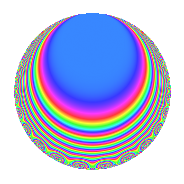# Properties

 Label 4032.2.b.oLevel 4032 Weight 2 Character orbit 4032.b Analytic conductor 32.196 Analytic rank 0 Dimension 8 CM No Inner twists 2

# Related objects

## Newspace parameters

 Level: $$N$$ = $$4032 = 2^{6} \cdot 3^{2} \cdot 7$$ Weight: $$k$$ = $$2$$ Character orbit: $$[\chi]$$ = 4032.b (of order $$2$$ and degree $$1$$)

## Newform invariants

 Self dual: No Analytic conductor: $$32.195682095$$ Analytic rank: $$0$$ Dimension: $$8$$ Coefficient field: 8.0.836829184.2 Coefficient ring: $$\Z[a_1, \ldots, a_{7}]$$ Coefficient ring index: $$2^{9}$$ Sato-Tate group: $\mathrm{SU}(2)[C_{2}]$

## $q$-expansion

Coefficients of the $$q$$-expansion are expressed in terms of a basis $$1,\beta_1,\ldots,\beta_{7}$$ for the coefficient ring described below. We also show the integral $$q$$-expansion of the trace form.

 $$f(q)$$ $$=$$ $$q -\beta_{1} q^{5} -\beta_{2} q^{7} +O(q^{10})$$ $$q -\beta_{1} q^{5} -\beta_{2} q^{7} + ( \beta_{2} + \beta_{4} + \beta_{7} ) q^{11} -\beta_{5} q^{13} + \beta_{1} q^{17} + ( 2 - \beta_{2} + \beta_{4} - \beta_{6} ) q^{19} + ( -\beta_{1} + \beta_{2} + \beta_{4} + \beta_{5} ) q^{23} + ( -2 + \beta_{3} + \beta_{6} ) q^{25} + ( -1 + \beta_{2} + \beta_{3} - \beta_{4} + \beta_{6} ) q^{29} + ( 2 + \beta_{6} ) q^{31} + ( 1 + \beta_{1} - \beta_{3} + \beta_{5} ) q^{35} + ( -1 + \beta_{3} - \beta_{6} ) q^{37} + ( \beta_{1} - 2 \beta_{5} ) q^{41} + ( \beta_{1} - \beta_{2} - \beta_{4} - 2 \beta_{5} - \beta_{7} ) q^{43} + ( 1 + \beta_{2} - \beta_{3} - \beta_{4} ) q^{47} + ( -1 - \beta_{1} + \beta_{2} - \beta_{4} - \beta_{5} + \beta_{6} + \beta_{7} ) q^{49} + ( 4 - 2 \beta_{2} + 2 \beta_{4} - \beta_{6} ) q^{53} + ( \beta_{2} + 2 \beta_{3} - \beta_{4} + \beta_{6} ) q^{55} + ( -3 - \beta_{2} - \beta_{3} + \beta_{4} ) q^{59} + ( -\beta_{5} - 2 \beta_{7} ) q^{61} + ( -1 - \beta_{2} + \beta_{3} + \beta_{4} ) q^{65} + ( 3 \beta_{1} - \beta_{2} - \beta_{4} - 2 \beta_{5} - \beta_{7} ) q^{67} + ( \beta_{1} + \beta_{2} + \beta_{4} + \beta_{5} ) q^{71} + ( 2 \beta_{1} - 2 \beta_{5} ) q^{73} + ( 4 + \beta_{1} - 2 \beta_{2} - 2 \beta_{4} - 2 \beta_{5} - \beta_{6} - 2 \beta_{7} ) q^{77} + ( -2 \beta_{1} - \beta_{2} - \beta_{4} - 2 \beta_{5} ) q^{79} + ( -5 + 3 \beta_{2} + \beta_{3} - 3 \beta_{4} ) q^{83} + ( 7 - \beta_{3} - \beta_{6} ) q^{85} + ( -\beta_{1} - 4 \beta_{2} - 4 \beta_{4} - 2 \beta_{5} ) q^{89} + ( 2 + \beta_{1} - \beta_{6} + \beta_{7} ) q^{91} + ( -4 \beta_{1} + 2 \beta_{5} + 2 \beta_{7} ) q^{95} + ( 2 \beta_{5} + 2 \beta_{7} ) q^{97} +O(q^{100})$$ $$\operatorname{Tr}(f)(q)$$ $$=$$ $$8q - 4q^{7} + O(q^{10})$$ $$8q - 4q^{7} + 8q^{19} - 16q^{25} + 16q^{31} + 8q^{35} - 8q^{37} + 16q^{47} + 16q^{53} + 8q^{55} - 32q^{59} - 16q^{65} + 32q^{77} - 16q^{83} + 56q^{85} + 16q^{91} + O(q^{100})$$

Basis of coefficient ring in terms of a root $$\nu$$ of $$x^{8} + 14 x^{6} + 61 x^{4} + 84 x^{2} + 4$$:

 $$\beta_{0}$$ $$=$$ $$1$$ $$\beta_{1}$$ $$=$$ $$($$$$\nu^{5} + 9 \nu^{3} + 16 \nu$$$$)/2$$ $$\beta_{2}$$ $$=$$ $$($$$$\nu^{6} + 11 \nu^{4} + 30 \nu^{2} + 4 \nu + 12$$$$)/4$$ $$\beta_{3}$$ $$=$$ $$($$$$-\nu^{6} - 9 \nu^{4} - 16 \nu^{2} + 2$$$$)/2$$ $$\beta_{4}$$ $$=$$ $$($$$$-\nu^{6} - 11 \nu^{4} - 30 \nu^{2} + 4 \nu - 12$$$$)/4$$ $$\beta_{5}$$ $$=$$ $$($$$$\nu^{5} + 11 \nu^{3} + 26 \nu$$$$)/2$$ $$\beta_{6}$$ $$=$$ $$($$$$-\nu^{6} - 11 \nu^{4} - 26 \nu^{2} + 4$$$$)/2$$ $$\beta_{7}$$ $$=$$ $$($$$$\nu^{7} + 13 \nu^{5} + 50 \nu^{3} + 54 \nu$$$$)/2$$
 $$1$$ $$=$$ $$\beta_0$$ $$\nu$$ $$=$$ $$($$$$\beta_{4} + \beta_{2}$$$$)/2$$ $$\nu^{2}$$ $$=$$ $$($$$$\beta_{6} - \beta_{4} + \beta_{2} - 8$$$$)/2$$ $$\nu^{3}$$ $$=$$ $$($$$$2 \beta_{5} - 5 \beta_{4} - 5 \beta_{2} - 2 \beta_{1}$$$$)/2$$ $$\nu^{4}$$ $$=$$ $$($$$$-7 \beta_{6} + 5 \beta_{4} + 2 \beta_{3} - 5 \beta_{2} + 42$$$$)/2$$ $$\nu^{5}$$ $$=$$ $$($$$$-18 \beta_{5} + 29 \beta_{4} + 29 \beta_{2} + 22 \beta_{1}$$$$)/2$$ $$\nu^{6}$$ $$=$$ $$($$$$47 \beta_{6} - 29 \beta_{4} - 22 \beta_{3} + 29 \beta_{2} - 246$$$$)/2$$ $$\nu^{7}$$ $$=$$ $$($$$$4 \beta_{7} + 134 \beta_{5} - 181 \beta_{4} - 181 \beta_{2} - 186 \beta_{1}$$$$)/2$$

## Character Values

We give the values of $$\chi$$ on generators for $$\left(\mathbb{Z}/4032\mathbb{Z}\right)^\times$$.

 $$n$$ $$127$$ $$577$$ $$1793$$ $$3781$$ $$\chi(n)$$ $$-1$$ $$-1$$ $$1$$ $$1$$

## Embeddings

For each embedding $$\iota_m$$ of the coefficient field, the values $$\iota_m(a_n)$$ are shown below.

For more information on an embedded modular form you can click on its label.

Label $$\iota_m(\nu)$$ $$a_{2}$$ $$a_{3}$$ $$a_{4}$$ $$a_{5}$$ $$a_{6}$$ $$a_{7}$$ $$a_{8}$$ $$a_{9}$$ $$a_{10}$$
3583.1
 − 2.06644i 2.63640i 0.222191i − 1.65222i 1.65222i − 0.222191i − 2.63640i 2.06644i
0 0 0 4.33660i 0 −1.65222 + 2.06644i 0 0 0
3583.2 0 0 0 2.31423i 0 0.222191 2.63640i 0 0 0
3583.3 0 0 0 1.72844i 0 −2.63640 0.222191i 0 0 0
3583.4 0 0 0 0.922382i 0 2.06644 + 1.65222i 0 0 0
3583.5 0 0 0 0.922382i 0 2.06644 1.65222i 0 0 0
3583.6 0 0 0 1.72844i 0 −2.63640 + 0.222191i 0 0 0
3583.7 0 0 0 2.31423i 0 0.222191 + 2.63640i 0 0 0
3583.8 0 0 0 4.33660i 0 −1.65222 2.06644i 0 0 0
 $$n$$: e.g. 2-40 or 990-1000 Embeddings: e.g. 1-3 or 3583.8 Significant digits: Format: Complex embeddings Normalized embeddings Satake parameters Satake angles

## Inner twists

Char. orbit Parity Mult. Self Twist Proved
1.a Even 1 trivial yes
28.d Even 1 yes

## Hecke kernels

This newform can be constructed as the intersection of the kernels of the following linear operators acting on $$S_{2}^{\mathrm{new}}(4032, [\chi])$$:

 $$T_{5}^{8} + 28 T_{5}^{6} + 196 T_{5}^{4} + 448 T_{5}^{2} + 256$$ $$T_{11}^{8} + 68 T_{11}^{6} + 1396 T_{11}^{4} + 10272 T_{11}^{2} + 18496$$ $$T_{19}^{4} - 4 T_{19}^{3} - 44 T_{19}^{2} + 96 T_{19} + 64$$